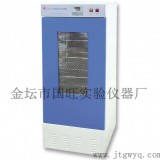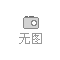•
••••# 150HL/250HL生化培养箱

• 面议
• 起订量：0
• 可售数量：0
• 发布时间：2018-01-05产品供应时间：长期有效

• 产品详情
• 联系方式
• 产品评价
• 品牌：
• 所属分类：实验室设备 » 制样/消解设备
• 计量单位：
• 最小起订量：0
• 供货总量：0
• 发货期限：自买家付款之日起3天内发货

 150HL/250HL生化培养箱价格的详细资料：
 生化培养箱 用途：  生化培养箱适用于细菌、霉菌、微生物、组织细胞的培育与保存；植物栽培、育种试验；酶学、酶工程研究；生物制品、药品、疫苗、血液和各种标本的保存与试验，是遗传工程、医学、农业、林业、环境科学、畜牧、水产等生产和科研部门的理想的实验设备。 生化培养箱 特点：  生化培养箱结构采用优质不锈钢内胆，中空玻璃，保温材料选用聚氨酯发泡，保温好、重量轻、整体外型美观，控制系统采用微电脑控制、数字显示，准确直观、精度高，温度调节方便可靠，有光照度便于观察。光照培养箱，光照可强可弱；恒温恒湿培养箱还可以恒湿。制冷系统为优质全封闭压缩机，制冷量大、噪音低，箱内配以优质风机装置，强迫空气对流，温度分布更加均匀。 生化培养箱 主要技术参数 ：   1.工作室容积:value="150" hasspace="False" negative="False" numbertype="1" tcsc="0">value="150" hasspace="False" negative="False" numbertype="1" tcsc="0">value="150" hasspace="False" negative="False" numbertype="1" tcsc="0">150升、value="250" hasspace="False" negative="False" numbertype="1" tcsc="0">value="250" hasspace="False" negative="False" numbertype="1" tcsc="0">value="250" hasspace="False" negative="False" numbertype="1" tcsc="0">250升  2.温度范围：5～value="50" hasspace="False" negative="False" numbertype="1" tcsc="0">value="50" hasspace="False" negative="False" numbertype="1" tcsc="0">value="50" hasspace="False" negative="False" numbertype="1" tcsc="0">50℃  3.温度均匀度：≤±value="1" hasspace="False" negative="False" numbertype="1" tcsc="0">value="1" hasspace="False" negative="False" numbertype="1" tcsc="0">value="1" hasspace="False" negative="False" numbertype="1" tcsc="0">1℃  4.温度波动度：≤±value="1" hasspace="False" negative="False" numbertype="1" tcsc="0">value="1" hasspace="False" negative="False" numbertype="1" tcsc="0">value="1" hasspace="False" negative="False" numbertype="1" tcsc="0">1℃  5.不锈钢内胆，喷塑钢板外壳，聚胺脂泡沫保温  6.设有大视角观察窗、照明灯  7.微电脑控制，数字显示精度高。  8.独特的不锈钢循环风道，强迫空气循环，温度均匀  生化培养箱 使用说明： 1、接通电源，合上电源开关，整机通电，开关内的电源指示灯亮。  2、控温设定请参照智能型数字温度控制器说明书。  3、如需照明打开照明开关。  4、本培养箱有断点保护功能及一分半钟左右延时功能，压缩机停机后再次启动要达一分半钟左右。  生化培养箱 维护和培养： 1、培养箱外壳应可靠接地。  2、培养箱要放置在阴凉、干燥、通风良好、远离热源和日晒的地方。放置平稳，以防震动发生噪音。  3、为保证冷凝器有效地散热，冷凝器与墙壁之间距离应大于value="100" hasspace="False" negative="False" numbertype="1" tcsc="0">value="100" hasspace="False" negative="False" numbertype="1" tcsc="0">100mm。箱体侧面应有value="50" hasspace="False" negative="False" numbertype="1" tcsc="0">value="50" hasspace="False" negative="False" numbertype="1" tcsc="0">50mm间隙，箱体顶部至少应有value="300" hasspace="False" negative="False" numbertype="1" tcsc="0">value="300" hasspace="False" negative="False" numbertype="1" tcsc="0">300mm空间。  4、培养箱在搬运、维修、保养时，应避免碰撞，摇晃震动；最大倾斜度小于45度。  5、仪器突然不工作，请检查熔丝管（箱后）是否烧坏，检查供电情况。  6、本生化培养箱制冷工作时，不宜使箱内温度与环境温度之差大于25度。 生化培养箱可根据顾客要求进行订做 生化培养箱 售后服务：三包一年，终身维修！

• 产品详情
• 联系方式
• 产品评价